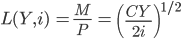# Demand for Money

The desired holding of financial assets in cash or bank deposits

Stephen Zarlenga defined money as the essence of an abstract social power embodied by law, as an unconditional means of payment.The demand represents the desire of households and businesses to hold assets in a form that can be easily exchanged for goods and services.

Liquidity is the key aspect of money that differentiates it from the other types of assets.

In monetary economics, the demand for money is the desired holding of financial assets in cash or bank deposits.

This is the sense that M1 is dominated as a store of value by interest-bearing assets where M1 is seen as directly spendable holdings. It is necessary for carrying out transactions, or we can also say that it provides liquidity.

Demand for M1 depends on the trade-off between the liquidity advantage of holding money for near future expenditures or the interest advantage of holding other financial assets regarding the form in which a person's spendable funds should be held.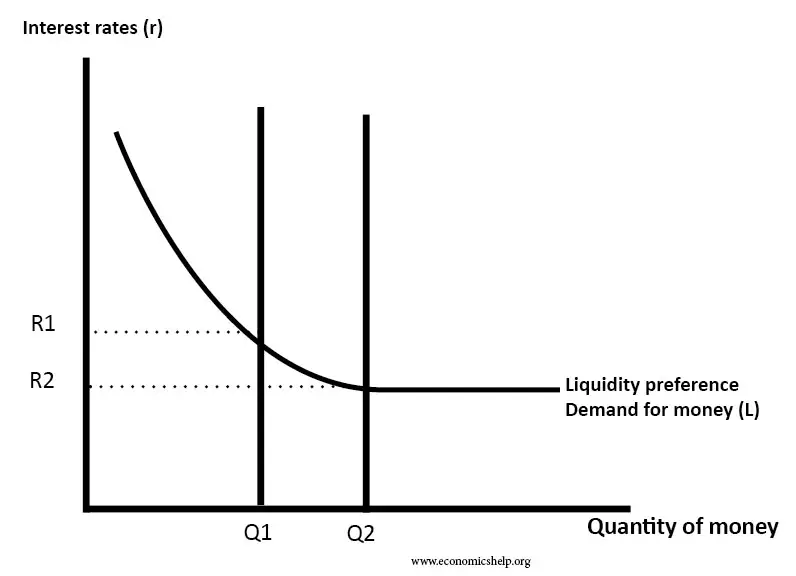The above diagram shows that its demand is inversely related to the interest rate.

• At high-interest rates, people prefer to hold bonds.

• At lower interest rates holding bonds give lower rates of return, so people prefer holding cash.

The demand is affected by various factors, including income level, interest rates, inflation, and uncertainties about the future. How these factors affect the demand can be explained in terms of various motives an individual has.

## Factors affecting demand for money

The factors affecting are:

1. Interest rate

• The amount people keep in reserves for transactions, meeting precautionary or speculative demand, varies with the interest rate they earn on the alternative assets.

• People are likely to hold less money when the interest rate rises relative to the rates available on the bonds and vice versa.

• The logic of the conclusion above is dependent on the motivations of people for holding money.

2. Technological changes

• The introduction of debit cards and online transfers using various methods marked the significance of cashless holding.

• People can keep less cash if they have easy access to current accounts.

• Less cash in hand is kept when new technologies make it easier to convert wealth into cash.3. Availability of Credit

With the wider availability of credits, precautionary demand falls, as people believe that whenever they face short-term difficulties, they can borrow easily.

4. Irrational Behavior of Asset Prices

• Psychological factors such as over-exuberance lead to markets going through busts and booms.

• Demand for assets rises during these bubble periods and holdings fall.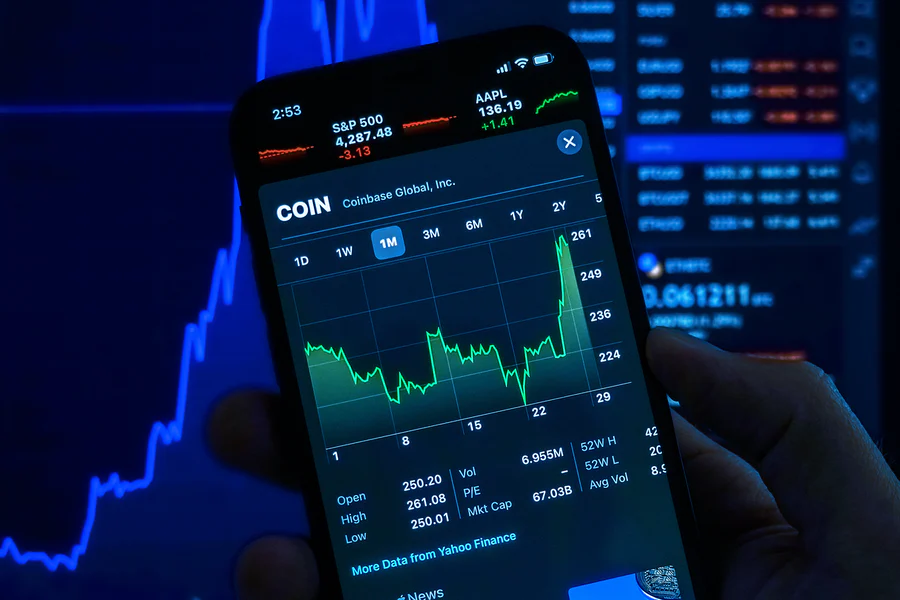5. Changes in National Income

More goods and services are available for purchase as real gross domestic product rises. They cost more to purchase as compared to the time when the real gross domestic product falls.

6. Changes in the Price Level

Changes in price level account for inflation or deflation. If the price of everything increases by 20%, an individual requires 20% more money to buy things which therefore leads to an increase in its demand.

## What are the motives for demanding money?

The motives behind are:

1. Transaction Motive

Transaction motive arises from the fact that mostly all the transactions require an exchange of money, and due to the necessity of availability, it is demanded in an economy massively.

The need for liquidity to meet day-to-day expenses arises as income received is an occasional event like per month, but the expenditures are continuously occurring events.

The number of transactions increases over time with an increase in income. This, in turn, means that an increase in Gross Domestic Product or income thereby increases the demand.2. Precautionary Motive

Since the future is highly uncertain and unpredictable, people need a certain amount to meet the sudden requirements. This comes under the precautionary motive of demand.

Unexpected expenses like medical bills, repair bills, or any other sudden expenditure need immediate payments, which requires holding the transaction funds.

3. Speculative Motive

Being an asset, its demand also depends on the rate of return and opportunity cost. The cash in hand provides no rate of return but depreciates over time with inflation.

The opportunity cost of holding money is the forgone interest rate one could have earned by lending or investing this money. The demand here, in turn, depends both on the current nominal interest rate and the expected future interest rate.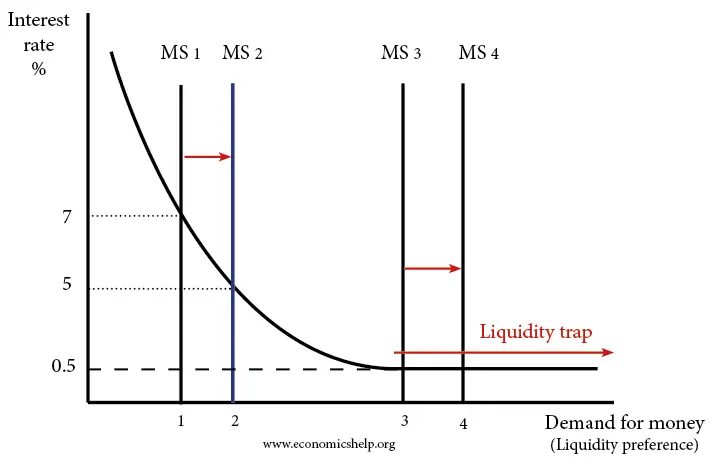Example: If a person expects the future interest rate to be lower than the current nominal interest rate, they will reduce their cash holdings and increase their bond holdings.

If the future interest rate falls, the price of the bond will increase, and the person will enjoy capital gains over their bond purchases.

If a person perceives these holdings as less risky than lending or investing them in some other assets, then an increase in speculative demand will be observed in the economy.

## The quantity theory of money

The quantity theory states that " the general price level of goods and services is directly proportional to the amount of money in circulation, or money supply."

The theory was originally formulated by Polish mathematician Nicolaus Copernicus in 1517 and became popular with economists Anna Schwartz and Milton Friedman's book A Monetary History of the United States, 1963.

1. Equation of exchange

Accordingly, behavioral interpretation can be given for the identity as demand for money is represented as Md

Also, M is its stock, V is the velocity, Y is real income, and PY is nominal income.

MV = PY

Md = P(Y/V)

(Md/P) = (Y/V)

This shows that demand is a function of price and income as long as the velocity is constant. Also, money demand is positively related to income and inversely related to price.2. Fisher's Equation of Exchange

In modern form, quantity theory builds upon the definitional relationship given by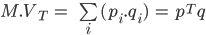• M is the total amount of money in circulation on average in an economy during a period.

• VT  is the transaction velocity of money. This reflects the average frequency across all transactions with which a unit of money is spent.

• pi and qare the price and quantity of the i-th transaction.

• p is the column vector of pi, and T stands for transpose of the vector.

• q is the column vector of qi.

A simplified version of the above equation is given as:

M.VT = PT.T

PT is the price level, and T is an index of the real value of aggregate transactions.

With the development of national income and product accounts, the emphasis shifted to national income or final product transactions. Here V becomes the velocity in final expenditure, and Q is an index of the real value of final expenditure.

Velocity is taken as the ratio of net national product in current prices to the money stock. The quantity theory postulates that the primary causal effect is an effect of M on P.

3. Cambridge approach

Economists Alfred Marshall, A.C. Pigou, and John Maynard Keynes are associated with Cambridge University. They focused on money's demand instead of its supply.

The portion of its supply is held in an economy for the convenience and security of having cash on hand. This portion is commonly represented as k, a portion of nominal income (P.Y).

The Cambridge equation is thus:

Md = k.P.Y

Assuming that the economy is at equilibrium (Md = M), Y is exogenous, and k is fixed in the short run.

M(1/k) = P.Y

Here,

(1/k) is the velocity.

4. Milton Friedman:

He emphasized the following relationships:

(i) PQ = f(M+)

(ii) P = g(M+)

The plus sign indicates that a change in the money supply is hypothesized to change nominal expenditures and the price level in the same direction.

The money multiplier indicates how much a change in banks' reserve ratio would change the supply.

MM = 1 ÷ RR

## Inventory model

A firm holds inventory so customers can buy. When the inventory is depleted, the firm replenishes the inventory.

Similarly, an individual holds an inventory of money to use for purchases. When the inventory is depleted, the individual replenishes the inventory.

The Baumol- Tobin model is an economic model whose theories are developed by William Baumol and James Tobin.

The theories laid by the two rely on "the trade-off between the liquidity provided by holding money and the interest forgone by holding one's assets in the form of non-interest bearing money."

The nominal interest rate, the level of the real income, and the fixed transaction costs are the key variables of demand here.

Also, the transaction cost is the cost of transferring one's wealth between interest-bearing assets and liquid assets, i.e., cash.According to Baumol, individuals have to keep an optimum inventory for their daily transaction purposes.

Cost is incurred at times they hold inventories, and the cost forgone is the interest rate that could have been earned by investing money.

Individuals compare the costs and benefits of the funds in the form of money with

(a) no interest

(b) in the form of savings deposits with some interest.

The average amount of cash withdrawals that minimizes the cost can be given by: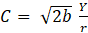This gives that square root of twice the broker's fee multiplied by the size of the individual's income and divided by the interest rate. This equation is also referred to as Square Root Rule.

Tobin emphasized the fact that, when individuals are faced with various safe and risky assets, they diversify their portfolio by holding a balanced combination of safe and risky assets.

Tobin believes that an individual's behavior shows risk aversion, which means they will not choose a portfolio that accounts for all risky bonds or a greater share of risky bonds even if the rate of return is high.

He derived his liquidity preference function showing the relationship between the rate of interest and demand for money. At a higher rate of interest, the demand for holding money will be less, and an individual will hold more bonds.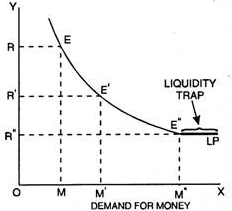In Tobin's portfolio approach, demand functions as asset slopes downwards, depicting that the demand will increase with a fall in the interest rate of bonds. Here, demand is shown on the x-axis, and the interest rate is shown on the y-axis.

Now for the formal exposition of the model

• Y is an individual's income which he spends at an even rate over some time and holds some portion of it to carry out transactions.

• C is the transaction cost per transfer which is fixed.

• N is the number of withdrawals.

• i is the nominal interest rate received at the end of the period.

The total cost of money management is given by:An optimal number of withdrawals is given by the derivative of the above expression concerning N, where N is set as N = 0.

Condition for optimum: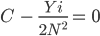This gives an optimal number of withdrawals as: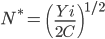Using average money holding equals to Y/2N, the demand function obtained looks like this: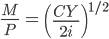The above equation can be modified to turn it into a demand for liquidity function